Reliable power for a sustainable world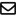info@rielloupsamerica.com
What Does Power Factor Mean With A UPS?

Power factor (pf) is the difference between actual energy consumed (Watts) and the apparent power (Volts multiplied by Amps) in an AC circuit. It is calculated as a decimal or percentage between 0-1 pf and 0-100% i.e. 0.9 pF = 90%.

The nearer the power factor is to unity (1 pf), the closer the two waveforms are in phase with each other and the device uses power more efficiently, hence why power factor relates to UPS efficiency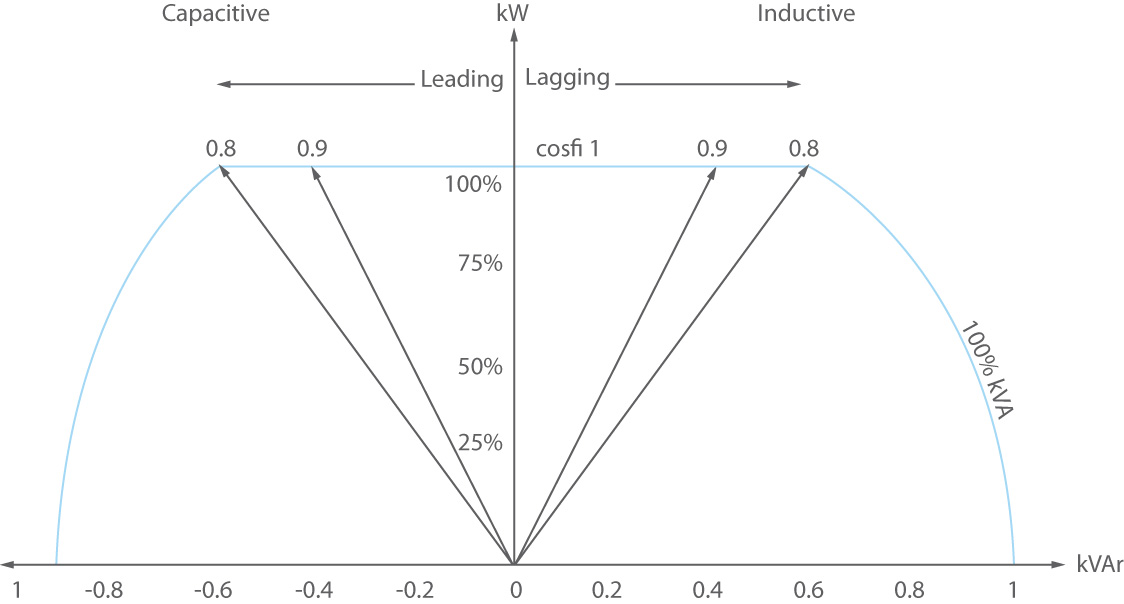Convention stipulates that inductive loads are defined as positive reactive power, with capacitive loads defined as negative reactive power. But power factor is never described as positive or negative, it is either lagging or leading.

### Lagging Power Factor

These are loads where the current waveform lags behind the voltage by a factor equal to the load’s reactance, typically between 0.5 and 0.95.

In the below image, a 2300 VA load with a lagging 0.766 pf would have a real power value of 1762 W (1.76 kW).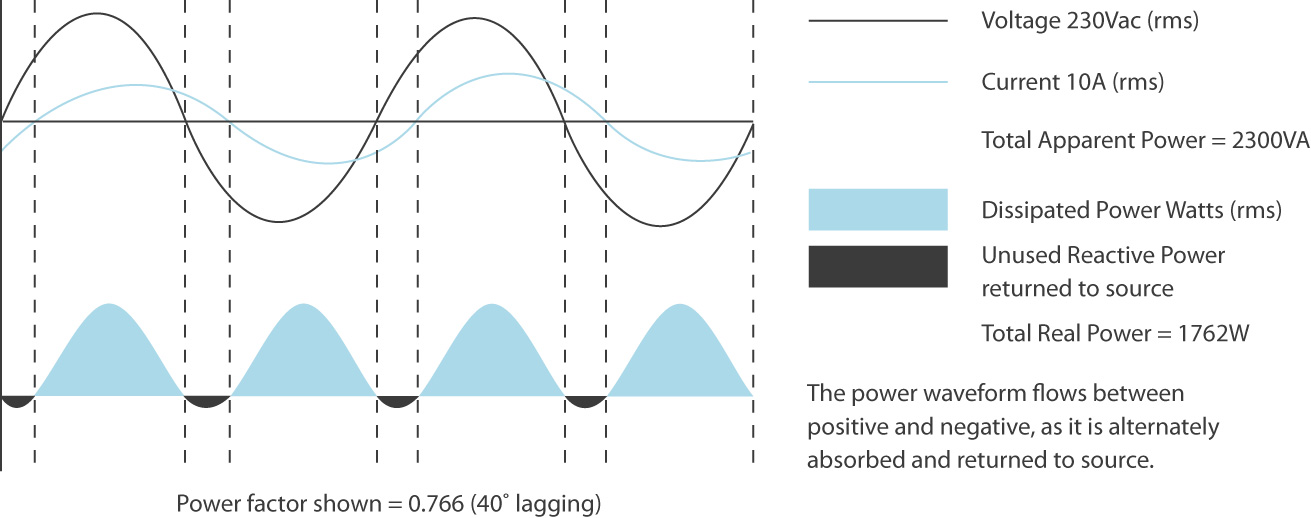### Unity Power Factor

Unity power factor (1 pf) loads have the current and voltage waveforms in phase with each other. In the example below, a 2300 VA load with 1 pf has a real power value of 2300 W (2.3 kW).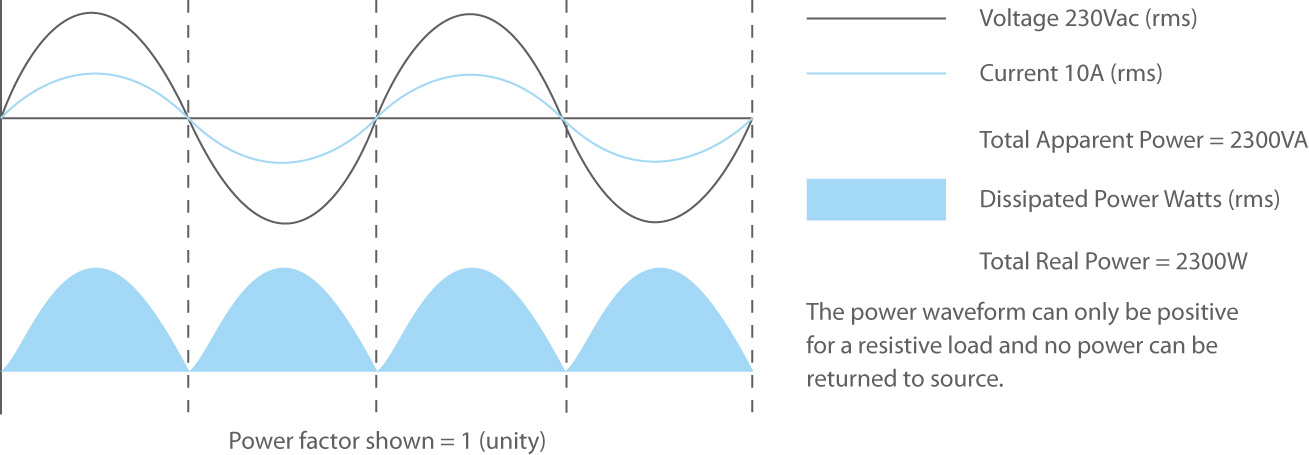### Leading Power Factor

Loads with a leading power factor have a current waveform that leads the voltage by a factor equal to the load’s reactance, usually between 0.8 and 0.95.

Using the same 2300 VA as in previous examples, a leading power factor of 0.766 has a real power value of 1762 W (1.76 kW).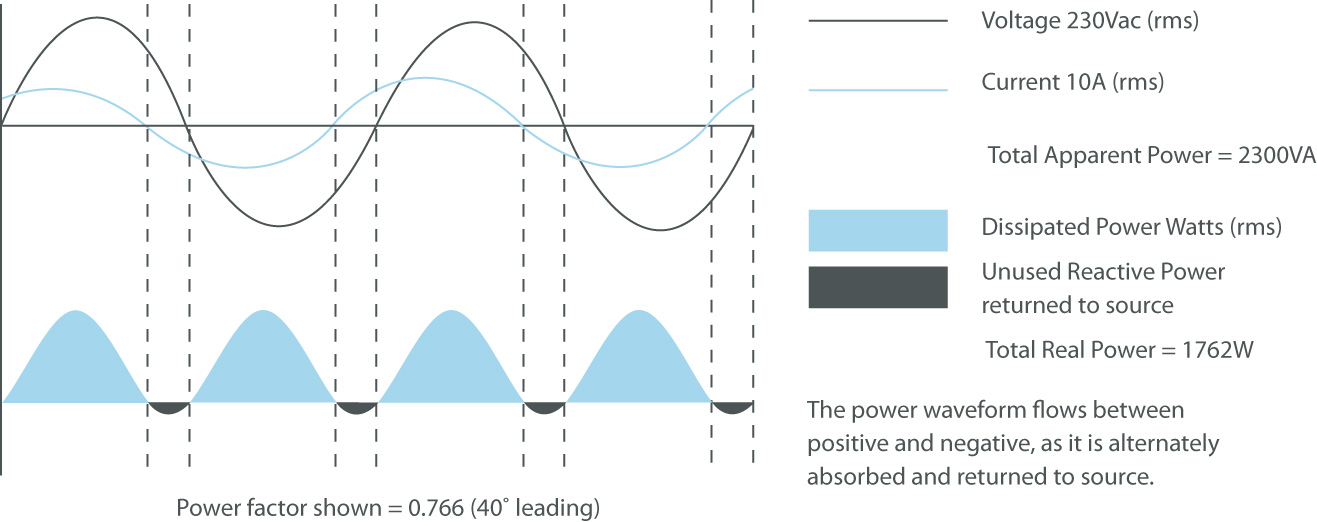### How Does Power Factor Influence UPS System Design?

Traditionally, UPS systems were designed to support loads with unity or lagging power factors.

However, modern uninterruptible power supplies can also now handle leading power factors. It does require careful planning during installation though, as leading power factors can place an overload on the UPS that it may not recognise.

Blade servers are the best example of a load with a leading power factor. They are capable of greater processing power within less rack space than traditional file servers and have been widely adopted in the telecoms and data centre sectors because of advantages such as simplified cabling and reduced power consumption.

There are several ways to try and reduce the impact of leading power factors, including increasing the size of the UPS, but the most common approach is to use active harmonic filters with power factor correction on the output.

This delivers a more acceptable load to the UPS, but it does reduce efficiency, take up more floor space and increase capital costs.